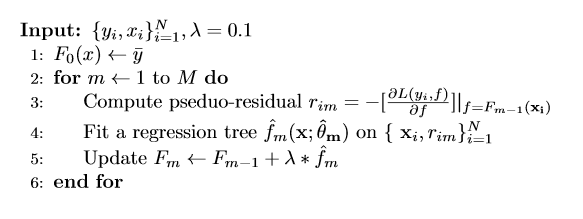## Abstract

In this tutorial, we train a Gradient Boosting Model (GBM) to forecast the intraday price movements of the SPY ETF using a collection of technical indicators. The implementation is based on the research produced by Zhou et al (2013), where a GBM was found to produce an annualized Sharpe ratio greater than 20. Our research shows that throughout a 5 year backtest, the model underperforms the SPY with its current parameter set. However, we finish the tutorial with highlighting potential areas of further research to improve the model’s performance.

## Background

A GBM is trained by setting the initial model prediction to the mean target value in the training set. The model then iteratively builds regression trees to predict the model’s pseudo-residuals on the training set to tighten the fit. The pseudo-residuals are the differences between the target value and the model’s prediction on the current training iteration for each sample. The model’s predictions are made by summing the mean target value and the products of the learning rate and the regression tree outputs. The full algorithm is shown here.We provide technical indicator values as inputs to the GBM. The model is trained to predict the security’s return over the next 10 minutes and the performance of the model’s predictions are assessed using the mean squared error loss function.

$MSE = \frac{\Sigma_{i=1}^n(y_i - \hat{y}_i)^2}{n}$

Zhou et al (2013) utilize custom loss functions to fit their GBM in a manner that aims to maximize the profit-and-loss or Sharpe ratio over the training data set. The attached notebook shows training the GBM with these custom loss functions leads to poor model predictions.

## Method

#### Universe Selection

We use a ManualUniverseSelectionModel to subscribe to the SPY ETF. The algorithm is designed to work with minute and second data resolutions. In our implementation, we use data on a minute resolution.

symbols = [ Symbol.Create("SPY", SecurityType.Equity, Market.USA) ]
self.SetUniverseSelection( ManualUniverseSelectionModel(symbols) )
self.UniverseSettings.Resolution = Resolution.Minute


#### Alpha Construction

The GradientBoostingAlphaModel predicts the direction of the SPY at each timestep. Each position taken is held for 10 minutes, although this duration is customizable in the constructor. During construction of this Alpha model, we simply set up a dictionary to hold a SymbolData object for each Symbol in the universe. In the case where the universe consists of multiple securities, the Alpha model holds each with equal weighting.

class GradientBoostingAlphaModel(AlphaModel):
symbol_data_by_symbol = {}

def __init__(self, hold_duration = 10):
self.hold_duration = hold_duration
self.weight = 1


#### Alpha Securities Management

When a new security is added to the universe, we create a SymbolData object for it to store information unique to the security. The management of the SymbolData objects occurs in the Alpha model's OnSecuritiesChanged method.

def OnSecuritiesChanged(self, algorithm, changes):
symbol = security.Symbol
self.symbol_data_by_symbol[symbol] = SymbolData(symbol, algorithm, self.hold_duration)

for security in changes.RemovedSecurities:
symbol_data = self.symbol_data_by_symbol.pop(security.Symbol, None)
if symbol_data:
symbol_data.dispose()

self.weight = 1 / len(self.symbol_data_by_symbol)


#### SymbolData Class

The SymbolData class is used in this algorithm to manage indicators, train the GBM, and produce trading predictions. The constructor definition is shown below. The class is designed to train at the end of each month, using the previous 4 weeks of data to fit the GBM that consists of 20 stumps (regression trees with 2 leaves). To ensure overnight holds are avoided, the class uses Scheduled Events to stop trading near the market close.

class SymbolData:
def __init__(self, symbol, algorithm, hold_duration, k_start=0.5, k_end=5,
k_step=0.25, training_weeks=4, max_depth=1, num_leaves=2, num_trees=20,
self.symbol = symbol
self.algorithm = algorithm
self.hold_duration = hold_duration
self.resolution = algorithm.UniverseSettings.Resolution
self.training_length = int(training_weeks * 5 * 6.5 * 60) # training_weeks in minutes
self.max_depth = max_depth
self.num_leaves = num_leaves
self.num_trees = num_trees

self.indicator_consolidators = []

# Train a model at the end of each month
self.model = None
algorithm.Train(algorithm.DateRules.MonthEnd(symbol),
algorithm.TimeRules.BeforeMarketClose(symbol),
self.train)

# Avoid overnight holds
self.allow_predictions = False
self.events = [
algorithm.Schedule.On(algorithm.DateRules.EveryDay(symbol),
algorithm.TimeRules.AfterMarketOpen(symbol, 0),
self.start_predicting),
algorithm.Schedule.On(algorithm.DateRules.EveryDay(symbol),
algorithm.TimeRules.BeforeMarketClose(symbol, hold_duration + 1),
self.stop_predicting)
]

self.setup_indicators(k_start, k_end, k_step)
self.train()


#### GBM Predictions

For brevity, we omit the model training logic. Although, the code can be seen in the attached backtest. To make predictions, we define the following method inside the SymbolData class. A position is held in the predicted direction only if the predicted return in that direction exceeds the cost of the trade.

def predict_direction(self):
if self.model is None or not self.allow_predictions:
return 0

input_data = [[]]
for _, indicators in self.indicators_by_indicator_type.items():
for indicator in indicators:
input_data.append(indicator.Current.Value)

return_prediction = self.model.predict(input_data)
if return_prediction > self.cost:
return 1
if return_prediction < -self.cost:
return -1
return 0


#### Alpha Update

As new TradeBars are provided to the Alpha model's Update method, each SymbolData object makes a directional prediction for its security. If the prediction is not flat, the Alpha model emits an insight in that direction with a duration of 10 minutes.

def Update(self, algorithm, data):
insights = []
for symbol, symbol_data in self.symbol_data_by_symbol.items():
direction = symbol_data.predict_direction()
if direction:
hold_duration = timedelta(minutes=self.hold_duration) # Should match universe resolution
insights.append(Insight.Price(symbol, hold_duration, direction, None, None, None, self.weight))

return insights


#### Portfolio Construction & Trade Execution

We utilize the InsightWeightingPortfolioConstructionModel and the ImmediateExecutionModel.

## Relative Performance

Period Name Start Date End Date Strategy Sharpe Variance
5 Year Backtest 9/1/2015 9/17/2020 Strategy -0.649 0.004
Benchmark 0.691 0.024
2020 Crash 2/19/2020 3/23/2020 Strategy -2.688 0.079
Benchmark -1.467 0.416
2020 Recovery 3/23/2020 6/8/2020 Strategy -2.083 0.019
Benchmark 7.942 0.101